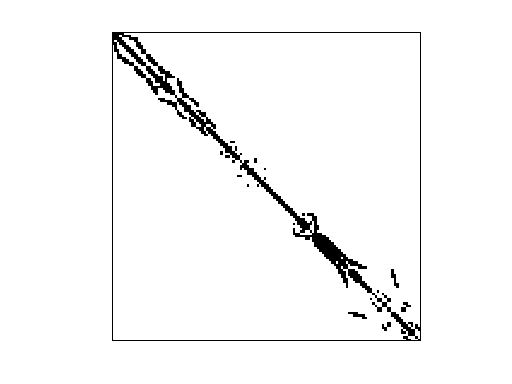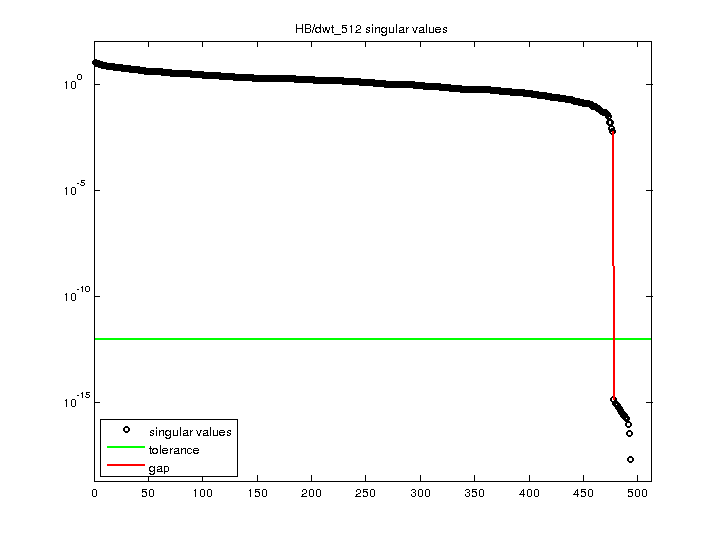Matrix: HB/dwt_512

Description: SYMMETRIC CONNECTION TABLE FROM DTNSRDC, WASHINGTON(undirected graph drawing)• Matrix group: HB
• download as a MATLAB mat-file, file size: 4 KB. Use UFget(128) or UFget('HB/dwt_512') in MATLAB.

 Matrix properties number of rows 512 number of columns 512 nonzeros 3,502 structural full rank? yes structural rank 512 # of blocks from dmperm 32 # strongly connected comp. 32 explicit zero entries 0 nonzero pattern symmetry symmetric numeric value symmetry symmetric type binary structure symmetric Cholesky candidate? yes positive definite? no

 author G. Everstine, D. Taylor editor I. Duff, R. Grimes, J. Lewis date 1980 kind structural problem 2D/3D problem? yes

 Ordering statistics: result nnz(chol(P*(A+A'+s*I)*P')) with AMD 3,759 Cholesky flop count 4.7e+04 nnz(L+U), no partial pivoting, with AMD 7,006 nnz(V) for QR, upper bound nnz(L) for LU, with COLAMD 4,696 nnz(R) for QR, upper bound nnz(U) for LU, with COLAMD 8,133

 SVD-based statistics: norm(A) 11.384 min(svd(A)) 0 cond(A) Inf rank(A) 477 sprank(A)-rank(A) 35 null space dimension 35 full numerical rank? no singular value gap 4.3133e+12

 singular values (MAT file): click here SVD method used: s = svd (full (A)) ; status: ok